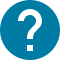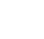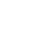学部後期課程
HOME 学部後期課程 数値シミュレーション技法

UTASにアクセスできない方は、担当教員または部局教務へお問い合わせ下さい。

# 数値シミュレーション技法

Mathematical modeling
In this course, students will learn the fundamentals of mathematical modeling.
A model is an idealized representation of the real world and mathematical modeling is the process by which the complexity of real-world phenomena is simplified such that they can be described, more abstractly, in mathematical terms. The hope is that this abstraction of the real world can then help us comprehend the essence of the phenomena we wish to understand and/or predict.
As such, the concept of mathematical modeling is common to virtually all empirical sciences—not only natural and engineering sciences but also human sciences such as economics, psychology, sociology, etc.

The lectures introduce several types of mathematical modeling strategies: optimization, modeling through dynamical systems (be they continuous, discrete or of cellular automaton-type) and, if time allows, probabilistic models.
The introductory part starts with the concept of optimization and the mathematical methods needed to tackle such problems. The middle and main part explores the behaviour of both discrete and continuous dynamical systems. The focus will lie on stability analysis for such systems as well as on the relationship between discrete and continuous models (continuum limits and discretisation methods). In the last part (if time permits), techniques for modeling probabilistic phenomena and stochastic processes as well as the concept of a Monte Carlo simulation will be introduced.

コース名

08D1420
FAS-DA4F20L3

WILLOX RALPH
A1 A2

2

NO

As the amount of time spent on a particular topic (and the amount of additional detail that might be given) will depend on the mathematical background of the students, there is no fixed lecture schedule. However, the lectures will cover: 1. Optimization concepts 2. Continuous time models 3. Stability analysis for continuous dynamical systems 4. Discrete time models and their relation to continuous models 5. Stability analysis of discrete dynamical systems 6. An introduction to cellular automata and, if there is sufficient time, 7. An introduction to probabilistic models and stochastic processes. A typical lecture will consist of: (a) an introduction of basic concepts and technical tools (b) worked out examples (c) in-class work in the form of exercises.

Lecture with in-class exercises. Circumstances allowing this course will be taught in-person, but it is not excluded that it could be moved online, occasionally, if the circumstances so require.

Grading is based on (1) class attendance (40%) (2) final report and class assignments (60%). No final examination.

There is no textbook for this course.

Hirsch, Morris W., Smale, Stephen, Devaney, Robert L., Differential Equations, Dynamical Systems & An Introduction to Chaos. Elsevier Academic Press 2004. Murray, James D., Mathematical Biology I: An Introduction. Springer 2002 (3rd Edition).

(1) Junior Division math Foundation Courses are prerequisite. (2) This course can be registered as one of the following titles, depending on the affiliation of the student. - Modeling and Simulation (required course for Environmental Sciences program) - Seminar in Global Liberal Arts II (21) Since part of the lecture will devoted to in class exercises, each lecture will be 105 minutes. In principle, all lectures will be in-person, including the lecture during the first week.